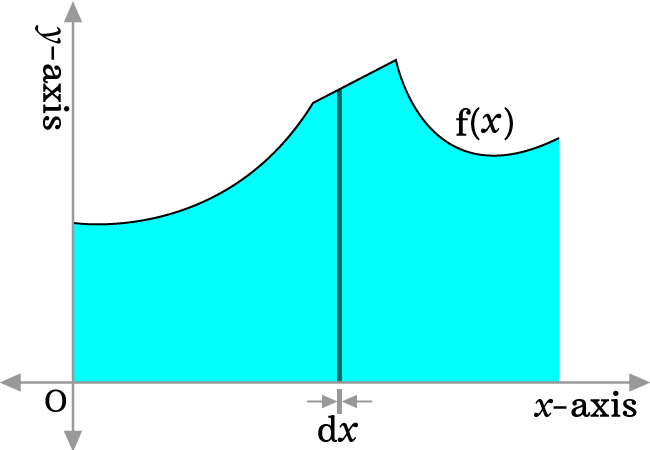# Indefinite integral calculus

A mathematical science that helps us to calculate the integral of a function without defining its interval is called the indefinite integral calculus.

## Introduction

The concept of calculating the indefinite integral of a function can be understood in calculus by constructing a graph geometrically.Let $f(x)$ be a mathematical function in terms of $x$. Construct a graph by representing the values of $x$ on the horizontal axis and the values of the function on the vertical axis. The area under the curve is denoted by sea-blue color (or colour).

In order to find the area under the curve, split the whole area into infinitely small equal parts. Now, consider a small part, which is denoted by a differential $dx$.

$\displaystyle \int{f(x)\,}dx$

### Integral rules

The list of the indefinite integration formulas with mathematical proofs.

### Problems

The list of indefinite integration questions with step by step procedure to learn how to find the indefinite integration of a function by using the integral rules.

Latest Math Topics
Jun 26, 2023
Jun 23, 2023

###### Math Questions

The math problems with solutions to learn how to solve a problem.

Learn solutions

Practice now

###### Math Videos

The math videos tutorials with visual graphics to learn every concept.

Watch now

###### Subscribe us

Get the latest math updates from the Math Doubts by subscribing us.